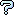All about flooble | fun stuff | Get a free chatterbox | Free JavaScript | Avatarsperplexus dot infoA Three Way Scale (Posted on 2005-09-05)In the tradition of weighing puzzles, you have yet another batch of coins to sort. The only way to distinguish the coins is by their weights. This time there are nine coins. Three coins weigh 20g, three coins weigh 21g, and three coins weigh 22g.

You need to sort the coins by weight, but this time you must use an unusual three way scale. The scale operates as follows:

- Three weights must be placed on the scale for a meaningful result.
- If all three weights are equal, the scale will be in perfect balance.
- If one weight is lighter than the other two, the scale will show that weight is the lightest.
- If two weights are equal and are lighter than the third, the scale will indicate that scenario.

Examples:
Ex1: If A=30 B=30 C=30 then the scale will be in balance.
Ex2: If A=30 B=31 C=32 then the scale will indicate that A is the lightest of the three, but not tell which of B and C is lighter.
Ex3: If A=30 B=31 C=31 then the scale will indicate that A is the lightest of the three, but not tell that B and C are equal. (undistinguishable from Ex2)
Ex4: If A=30 B=30 C=31 then the scale will indicate that A and B are equal and less than C.

Using this three way scale, can you sort the coins in five weighings?

 See The Solution Submitted by Brian Smith Rating: 4.0000 (1 votes)Subject Author Datere: Is 4 weighings possible? james 2005-09-13 09:40:49Is 4 weighings possible? Charlie 2005-09-06 13:48:19a lengthy solution Paul 2005-09-06 02:26:36Please log in:

 Search: Search body:
Forums (2)